# User:IssaRice/Computability and logic/Models symbol

The "models" symbol,$\models$, is used for several purposes in mathematical logic. Roughly, there are two basic purposes:

1. When the symbol that comes before "$\models$" is a structure/interpretation, then it says something about truth in that structure/interpretation.
2. When the symbol that comes before "$\models$" is a sentence or set of sentences, then it says something about semantic consequence (also called logical consequence, logical implication, semantic implication). In this case, we are talking about all possible structures/interpretations.

In either of the above two purposes, we are talking about the semantics (rather than syntax) of a logical system.

• If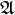$\mathfrak A$ is a structure/interpretation and$\Gamma$ is a set of sentences, then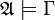$\mathfrak A \models \Gamma$ means ...
• If$T$ is a theory and$\phi$ is a sentence, then$T \models \phi$ means ...
• If$T$ is a theory and$\Gamma$ is a set of sentences, then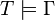$T \models \Gamma$ means ...
• If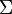$\Sigma$ is a set of axioms for a theory$T$, and$\phi$ is a sentence, then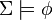$\Sigma \models \phi$ means ...
• If$\Sigma$ is a set of axioms for a theory$T$, and$\Gamma$ is a set of sentences, then ...
• if$\phi$ is a formula (or wff), then ...
• also the variant without anything in front, e.g.,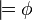$\models \phi$
Part before "$\models$"$\models$ Part after "$\models$" Possible pronunciations Meaning
A structure/interpretation$\mathfrak A$$\models$ A sentence or formula$\phi$ The structure$\mathfrak A$ satisfies the formula$\phi$.
The formula$\phi$ is true in$\mathfrak A$.
The structure$\mathfrak A$ makes true the formula$\phi$.
A set of sentences or formulas$\Gamma$$\models$ A sentence or formula$\phi$$\phi$ is a logical consequence of$\Gamma$.$\Gamma$ logically implies$\phi$.$\phi$ is a semantic consequence of$\Gamma$.$\phi$ is true in every model of$\Gamma$.
Nothing$\models$ A sentence or formula$\phi$$\phi$ is valid.$\phi$ is a tautology (especially in the case of propositional logic).